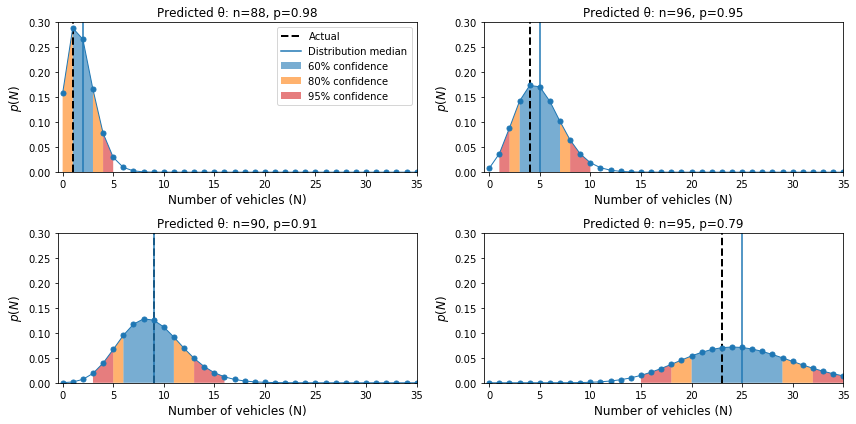# A guide to generating probability distributions with neural networks

Source: Deep Learning on Medium# A guide to generating probability distributions with neural networks

A few months ago we published an article that introduced the concept of confidence intervals and showed how, by sampling “thinned” networks using dropout, we go about generating them for pre-trained neural network regression models.

What if instead, as suggested by some of the readers, we wanted to train a model that can predict its own (un)certainty? In this article we will show you how we do just that, using Tensorflow with the Keras functional API to train a neural network that predicts a probability distribution for the target variable.

We’ll be using the exact same dataset as the first article — traffic flow — and modelling the target variable with a negative binomial distribution. However, I’m going to try and explain each step in a general manner, so that hopefully you can extend the process to any dataset and distribution of your choosing.

# Choosing a probability distribution

To train a model that predicts a probability distribution, we must first decide on what general shape the distribution will take. There are a lot of possible distributions to choose from, but that choice will be in part determined by any constraints on the values the target variable is allowed to take. Is the target discrete or continuous? Does it have fixed upper and lower limits, or an infinite range?

Let’s take a few examples from the kind of projects we often work on at HAL24K.

Water levels are continuous and effectively unbounded (within the region of probable values). For these we use a Gaussian distribution.